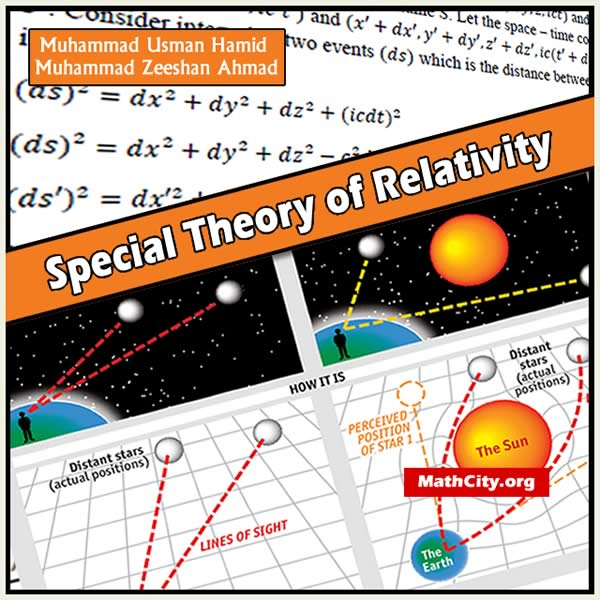# Special Theory of Relativity by M Usman Hamid and M Zeeshan AhmadSpecial theory of relativity developed by Einstein in 1905, after the failure of Newton‘s laws, when he was dealing with the relative motion in space. The theory of relativity deals with relations which exist between physical quantities (such as mass of a particle, length of a rod, electric field at a point etc.) as they appear to different observers in relative motion. The observers considered in this book are restricted to those in inertial frames of reference. The theory is then called restricted theory or Special Theory of Relativity (STR). When no such restriction is made, the theory is called the General Theory of Relativity. In 1915, Einstein developed General Theory of Relativity.

We are really very thankful to Muhammad Usman Hamid for providing these notes and appreciates his effort to publish these notes on MathCity.org

 Name Special Theory of Relativity Muhammad Usman Hamid and Muhammad Zeeshan Ahmad 170 pages PDF (see Software section for PDF Reader) 6.72 MB
• Galilean Transformation (G.T) / Newtonian Transformation
• Postulates Of Special Theory Of Relativity
• Michelson Morley Experiment (1887)
• Lorentz Transformations / Restricted Galilean Transformations /3d Lorentz Transformations
• Inverse Lorentz Transformations
• Length Contraction / Space Contraction /Lorentz Contraction
• Time Dilation
• Reciprocity Between Observers
• Inverse Law Of Transformation Of Velocities
• Composition Of Acceleration / Lorentz Transformation Law Of Acceleration
• Inverse Lorentz Transformation Law Of Acceleration
• Proper Time Interval / Proper Time / World Time
• Rotation Of Axes
• Rapidly Or Pseudo Velocity
• General Lorentz Transformations Fixed Without Rotation / Restricted Lorentz Transformations
• General Inverse Lorentz Transformations Fixed Without Rotation / Restricted Inverse Lorentz Transformations
• Four-Vector
• Four–Velocity / Minkowski Velocity
• Transformation Law Of 4–Velocity
• Four–Momentum / Minkowski Momentum
• Transformation Law Of 4-Momentum
• Four–Force / Minkowski Force
• Transformation Law Of 4-Momentum
• Mass Energy Relationship In Terms Of Str
• Equivalence Of Mass And Energy (Or Proof Of $E-mc^2$)
• Relativistic Energy /Momentum Energy Relation
• Mass Energy Relationship In Terms Of Classical Mechanics
• Mass Energy Relationship In Terms Of $\gamma(u)$
• Relativity Of Mass / Mass Dilation Problem
• Interval Between Events
• Timelike Intervals
• Null Intervals
• Spacelike Intervals
• Light Cone / Null Cone
• Causality
• Compton Effect
• Doppler Effect / Doppler Shift
• Aberration Of Light